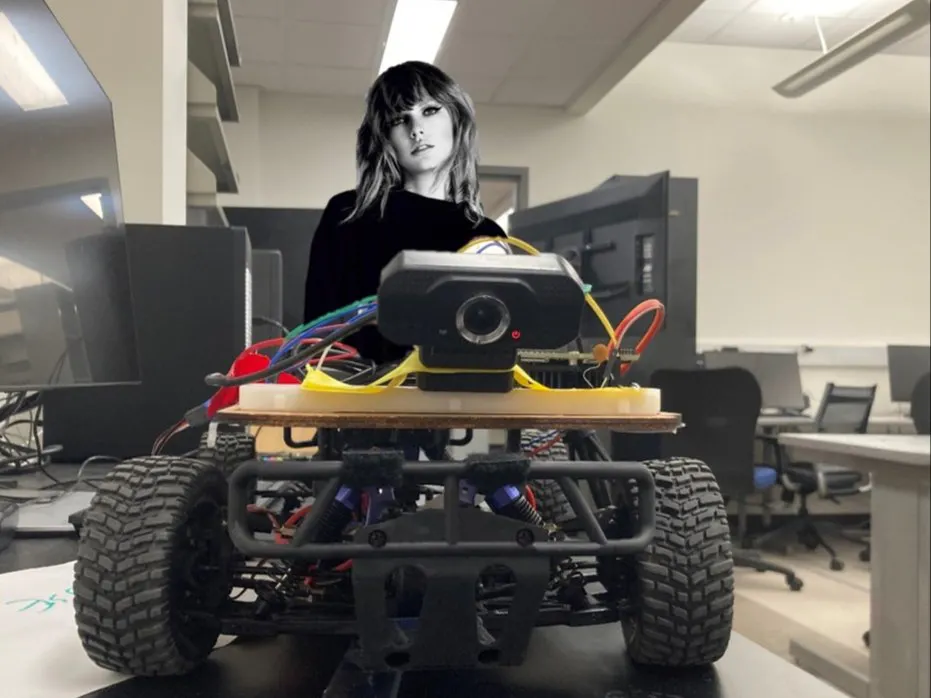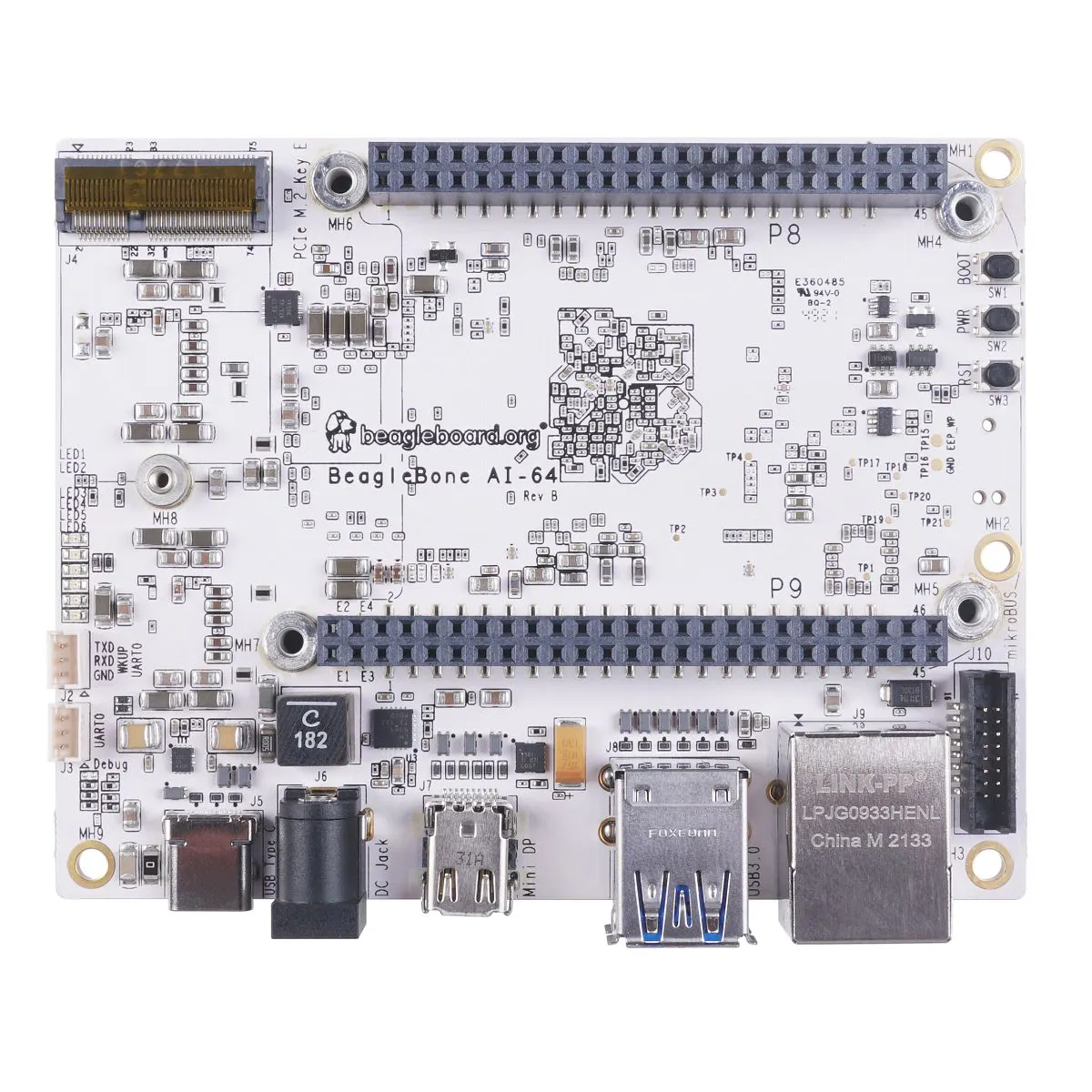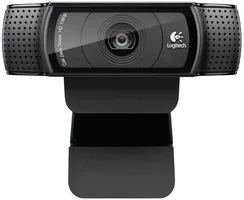Published

# BBL Autonomous RC Getaway Car

An autonomous vehicle on a BBAI-64 that's capable of lane-keeping and stopping at a stop box (good for getaways).

IntermediateShowcase (no instructions)252## Things used in this project

### Hardware componentsBeagleBoard.org BeagleBone AI-64
×1Webcam, Logitech® HD Pro
×1
 Portable Charger
×1
×1

### Software apps and online servicesOpenCV

## Code

### Video Processing Functions

Python
Functions to take camera feed and filter out the necessary info to detect where the car is position relative to the blue boundary lines and turn accordingly.
```import cv2
import matplotlib.pylot as plt
import numpy as np

def getRedFloorBoundaries():
"""
Gets the hsv boundaries and success boundaries indicating if the floor is red
:return: [[lower color and success boundaries for red floor], [upper color and success boundaries for red floor]]
"""
return getBoundaries("redboundaries.txt")

def isRedFloorVisible(frame):
"""
Detects whether or not the floor is red
:param frame: Image
:return: [(True is the camera sees a red on the floor, false otherwise), video output]
"""
print("Checking for floor stop")
boundaries = getRedFloorBoundaries()
return isMostlyColor(frame, boundaries)

def getTrafficRedLightBoundaries():
"""
Gets the traffic red light hsv boundaries and success boundaries
:return: [[lower color and success boundaries for red light], [upper color and success boundaries for red light]]
"""
return getBoundaries("trafficRedBoundaries.txt")

def isTrafficRedLightVisible(frame):
"""
Detects whether or not we can see a stop sign
:param frame:
:return: [(True is the camera sees a stop light, false otherwise), video output]
"""
print("Checking for traffic stop")
boundaries = getTrafficRedLightBoundaries()
return isMostlyColor(frame, boundaries)

def getTrafficGreenLightBoundaries():
"""
Gets the traffic green light hsv boundaries and success boundaries
:return: [[lower color and success boundaries for green light], [upper color and success boundaries for green light]]
"""
return getBoundaries("trafficGreenboundaries.txt")

def isTrafficGreenLightVisible(frame):
"""
Detects whether or not we can see a green traffic light
:param frame:
:return: [(True is the camera sees a green light, false otherwise), video output]
"""
print("Checking For Green Light")
boundaries = getTrafficGreenLightBoundaries()
return isMostlyColor(frame, boundaries)

def isMostlyColor(image, boundaries):
"""
Detects whether or not the majority of a color on the screen is a particular color
:param image:
:param boundaries: [[color boundaries], [success boundaries]]
:return: boolean if image satisfies provided boundaries, and an image used for debugging
"""
#Convert to HSV color space
hsv_img = cv2.cvtColor(image, cv2.COLOR_BGR2HSV)

#parse out the color boundaries and the success boundaries
color_boundaries = boundaries
percentage = boundaries

lower = np.array(color_boundaries)
upper = np.array(color_boundaries)

#Calculate what percentage of image falls between color boundaries
print("percentage_detected " + str(percentage_detected) + " lower " + str(lower) + " upper " + str(upper))
# If the percentage percentage_detected is betweeen the success boundaries, we return true, otherwise false for result
result = percentage < percentage_detected <= percentage
if result:
print(percentage_detected)
return result, output

def getBoundaries(filename):
"""
Reads the boundaries from the file filename
Format:
 lower: [H, S, V, lower percentage for classification of success]
 upper: [H, S, V, upper percentage for classification of success]
:param filename: file containing boundary information as above
:return: [[lower color and success boundaries], [upper color and success boundaries]]
"""
default_lower_percent = 50
default_upper_percent = 100
with open(filename, "r") as f:
lower_data = [val for val in boundaries.split(",")]
upper_data = [val for val in boundaries.split(",")]

if len(lower_data) >= 4:
lower_percent = float(lower_data)
else:
lower_percent = default_lower_percent

if len(upper_data) >= 4:
upper_percent = float(upper_data)
else:
upper_percent = default_upper_percent

lower = [int(x) for x in lower_data[:3]]
upper = [int(x) for x in upper_data[:3]]
boundaries = [lower, upper]
percentages = [lower_percent, upper_percent]
return boundaries, percentages

def detect_edges(frame):
# filter for blue lane lines
hsv = cv2.cvtColor(frame, cv2.COLOR_BGR2HSV)
# cv2.imshow("HSV",hsv)
lower_blue = np.array([90, 120, 0], dtype="uint8")
upper_blue = np.array([150, 255, 255], dtype="uint8")

# detect edges
# cv2.imshow("edges",edges)

return edges

def region_of_interest(edges):
height, width = edges.shape

# only focus lower half of the screen
polygon = np.array([[
(0, height),
(0, height / 2),
(width, height / 2),
(width, height),
]], np.int32)

# cv2.imshow("roi",cropped_edges)

return cropped_edges

def detect_line_segments(cropped_edges):
rho = 1
theta = np.pi / 180
min_threshold = 10

line_segments = cv2.HoughLinesP(cropped_edges, rho, theta, min_threshold,
np.array([]), minLineLength=5, maxLineGap=150)

return line_segments

def average_slope_intercept(frame, line_segments):
lane_lines = []

if line_segments is None:
print("no line segments detected")
return lane_lines

height, width, _ = frame.shape
left_fit = []
right_fit = []

boundary = 1 / 3
left_region_boundary = width * (1 - boundary)
right_region_boundary = width * boundary

for line_segment in line_segments:
for x1, y1, x2, y2 in line_segment:
if x1 == x2:
print("skipping vertical lines (slope = infinity")
continue

fit = np.polyfit((x1, x2), (y1, y2), 1)
slope = (y2 - y1) / (x2 - x1)
intercept = y1 - (slope * x1)

if slope < 0:
if x1 < left_region_boundary and x2 < left_region_boundary:
left_fit.append((slope, intercept))
else:
if x1 > right_region_boundary and x2 > right_region_boundary:
right_fit.append((slope, intercept))

left_fit_average = np.average(left_fit, axis=0)
if len(left_fit) > 0:
lane_lines.append(make_points(frame, left_fit_average))

right_fit_average = np.average(right_fit, axis=0)
if len(right_fit) > 0:
lane_lines.append(make_points(frame, right_fit_average))

return lane_lines

def make_points(frame, line):
height, width, _ = frame.shape

slope, intercept = line

y1 = height  # bottom of the frame
y2 = int(y1 / 2)  # make points from middle of the frame down

if slope == 0:
slope = 0.1

x1 = int((y1 - intercept) / slope)
x2 = int((y2 - intercept) / slope)

return [[x1, y1, x2, y2]]

def display_lines(frame, lines, line_color=(0, 255, 0), line_width=6):
line_image = np.zeros_like(frame)

if lines is not None:
for line in lines:
for x1, y1, x2, y2 in line:
cv2.line(line_image, (x1, y1), (x2, y2), line_color, line_width)

line_image = cv2.addWeighted(frame, 0.8, line_image, 1, 1)

return line_image

def display_heading_line(frame, steering_angle, line_color=(0, 0, 255), line_width=5):
height, width, _ = frame.shape

steering_angle_radian = steering_angle / 180.0 * math.pi

x1 = int(width / 2)
y1 = height
x2 = int(x1 - height / 2 / math.tan(steering_angle_radian))
y2 = int(height / 2)

cv2.line(heading_image, (x1, y1), (x2, y2), line_color, line_width)

def get_steering_angle(frame, lane_lines):
height, width, _ = frame.shape

if len(lane_lines) == 2:
_, _, left_x2, _ = lane_lines
_, _, right_x2, _ = lane_lines
mid = int(width / 2)
x_offset = (left_x2 + right_x2) / 2 - mid
y_offset = int(height / 2)

elif len(lane_lines) == 1:
x1, _, x2, _ = lane_lines
x_offset = x2 - x1
y_offset = int(height / 2)

elif len(lane_lines) == 0:
x_offset = 0
y_offset = int(height / 2)

angle_to_mid_deg = int(angle_to_mid_radian * 180.0 / math.pi)
steering_angle = angle_to_mid_deg + 90

return steering_angle

def plot_pd(p_vals, d_vals, error, show_img=False):
fig, ax1 = plt.subplots()
t_ax = np.arange(len(p_vals))
ax1.plot(t_ax, p_vals, '-', label="P values")
ax1.plot(t_ax, d_vals, '-', label="D values")
ax2 = ax1.twinx()
ax2.plot(t_ax, error, '--r', label="Error")

ax1.set_xlabel("Frames")
ax1.set_ylabel("PD Value")
ax2.set_ylim(-90, 90)
ax2.set_ylabel("Error Value")

plt.title("PD Values over time")
fig.legend()
fig.tight_layout()
plt.savefig("pd_plot.png")

if show_img:
plt.show()
plt.clf()

def plot_pwm(speed_pwms, turn_pwms, error, show_img=False):
fig, ax1 = plt.subplots()
t_ax = np.arange(len(speed_pwms))
ax1.plot(t_ax, speed_pwms, '-', label="Speed PWM")
ax1.plot(t_ax, turn_pwms, '-', label="Steering PWM")
ax2 = ax1.twinx()
ax2.plot(t_ax, error, '--r', label="Error")

ax1.set_xlabel("Frames")
ax1.set_ylabel("PWM Values")
ax2.set_ylabel("Error Value")

plt.title("PWM Values over time")
fig.legend()
plt.savefig("pwm_plot.png")

if show_img:
plt.show()
plt.clf()
```

### Lane Detection

Python
Code for detecting blue boundary lines from the camera and adjusting turn amount to stay within the lines.
```import cv2
import time
import math
import sys
import os
import matplotlib.pyplot as plt
from video_functions import *

#### Has no motor control, only will print steering

### PWM and Throttle
# Throttle
go_forward = 1637000
#dont_move = 1550000

# Speed and turning
stop = False
current_speed = go_forward
left = 9
right = 6

# set up video
video = cv2.VideoCapture(2)
video.set(cv2.CAP_PROP_FRAME_WIDTH, 320)
video.set(cv2.CAP_PROP_FRAME_HEIGHT, 240)

# wait for video to load
time.sleep(1)

# counter for number of ticks
counter = 0
max_ticks = 2000
stopSignCheck = 2
passedFirstStopSign = False
isStopSign = False

# PD variables

### Changing these
kp = 0.05
kd = kp * 0.10
####
lastTime = 0
lastError = 0

# arrays for making the final graphs
p_vals = []
d_vals = []
err_vals = []
speed_pwm = []
steer_pwm = []
encoder_vals =[]
hit_end = False

def stop():
stop = True
with open('/dev/bone/pwm/1/a/duty_cycle', 'w') as filetowrite:
filetowrite.write("1550000")

def go():
stop = False
with open('/dev/bone/pwm/1/a/duty_cycle', 'w') as filetowrite:
filetowrite.write(str(go_forward))

# Go!!
go()

while counter < max_ticks:
print("encoder vals: ", encoder_vals)
frame = cv2.resize(original_frame, (160, 120))

# Check for stop sign every couple ticks
if ((counter + 1) % stopSignCheck) == 0:
# Check for the first stop sign on the floor
if not passedFirstStopSign:
isStopSign, floorSight = isRedFloorVisible(frame)
# Vision debugging: will show anything red
#cv2.imshow("floorSight", floorSight)
#cv2.waitKey(1)
# We see a stop sign
if isStopSign:
print("-----------STOP SIGN DETECTED!!!!-----------")
# Stop the car after moving forward a little bit
# The stop is detected before the car arrives to the sign
time.sleep(0.1)
stop()
time.sleep(2)
passedFirstStopSign = True
# # This is used to not check for the second stop sign until many frames later
secondStopSignTick = counter + 200
# Check for a stop sign less frequently
stopSignCheck = 1
# Go faster so we can make the turn
go_forward = 1644000
go()

# Check for the stop sign at the end
elif passedFirstStopSign and counter > secondStopSignTick:
isStopSign2, _ = isRedFloorVisible(frame)
if isStopSign2:
print("------LAST STOP SIGN DETECTED!!!!------")
time.sleep(1)
# Stop the car
stop()
break

# Process the frame to figure out the right steering angle
edges = detect_edges(frame)
roi = region_of_interest(edges)
line_segments = detect_line_segments(roi)
lane_lines = average_slope_intercept(frame, line_segments)
lane_lines_image = display_lines(frame, lane_lines)
steering_angle = get_steering_angle(frame, lane_lines)

# Video processing debugging
#cv2.waitKey(0)

# Encoder logic; keep a good speed
with open('/var/log/kern.log') as f:
lastline = lines[len(lines)-1]
array = lastline.split()
if(array=="between:"):
encoderval = int(array)
encoder_vals.append(encoderval)
#print(encoderval)
if (1000 < encoderval < 20000000):
go_forward = str(int(go_forward) - 500)
elif (encoderval > 23000000):
go_forward = str(int(go_forward) + 500)

go()

#PD controller
now = time.time()
dt = now - lastTime

deviation = steering_angle - 90
error = abs(deviation)
steering = 0
derivative = kd * (error - lastError) / dt
proportional = kp * error
PD = int(steering + derivative + proportional)
steering = abs(PD)
print("steering: " + str(steering))

# Take values for graphs
p_vals.append(proportional)
d_vals.append(derivative)
err_vals.append(error)

#steering logic using PD values
new_val = 7.5
if deviation < 9 and deviation > -9:
print("Not Steering")
print("Deviation: " + str(deviation))
new_val = 7.5
deviation = 0
error = 0

elif deviation > 15:
print("Steering Right")
print("Deviation: " + str(deviation))
new_val = 6 - steering/90
print("right val: " + str(new_val))

elif deviation > 9:
print("Steering right")
print("Deviation: " + str(deviation))
new_val = 6 - steering/70
print("right val: " + str(new_val))

elif deviation < -25:
print("Steering Left")
print("Deviation: " + str(deviation))
new_val = 8.6 + steering/50
print("left val: " + str(new_val))

elif deviation < -20:
print("Steering Left")
print("Deviation: " + str(deviation))
new_val = 8.6 + steering/60
print("left val: " + str(new_val))

elif deviation < -9:
print("Steering Left")
print("Deviation: " + str(deviation))
new_val = 8.1 + steering/60
print("left val: " + str(new_val))

# Convert the PWM percentage value in terms of the actual PWM
# relative to 1500000 (base)

# Tell the beaglebone to turn
with open('/dev/bone/pwm/1/b/duty_cycle', 'w') as filetowrite:

# take values for graphs
speed_pwm.append(current_speed)

# update PD values for next loop
lastError = error
lastTime = time.time()

counter += 1

# clean up resources
video.release()
cv2.destroyAllWindows()
# Might have to change these to the right function
with open('/dev/bone/pwm/1/a/duty_cycle', 'w') as filetowrite:
filetowrite.write('1550000')
with open('/dev/bone/pwm/1/b/duty_cycle', 'w') as filetowrite:
filetowrite.write('1500000')

plot_pd(p_vals, d_vals, err_vals, True)
plot_pwm(speed_pwm, steer_pwm, err_vals, True)
```

### Encoder Module

C/C++
Kernel module for getting values from the encoder
```#include <linux/module.h>
#include <linux/of_device.h>
#include <linux/kernel.h>
#include <linux/gpio/consumer.h>
#include <linux/platform_device.h>
#include <linux/interrupt.h>
#include <linux/init.h>
#include <linux/ktime.h>
#include <linux/unistd.h>
//Initialize IRQ number and encodergpiodesc
unsigned int irq_number;
struct gpio_desc *encodergpio;
ktime_t start, end, elapsed_time;
//Ktime values to track encoder duration
//IRQ handler which records time since last ISR and prints it to kernel log to be read by the driving code
static irq_handler_t gpio_irq_handler(unsigned int irq, void *dev_id, struct
pt_regs *regs) {
end = ktime_get();
elapsed_time = ktime_sub(end,start);
start = ktime_get();
printk("Encoder has ticked, time between: %llu \n",elapsed_time);
return (irq_handler_t) IRQ_HANDLED;
}
// probe function
static int led_probe(struct platform_device *pdev)
{
//Start first time interval upon init
start = ktime_get();
//Define encodergpio
encodergpio = devm_gpiod_get(&pdev->dev, "userbutton", GPIOD_IN);
//debounce encoder input
gpiod_set_debounce(encodergpio, 1000000);
//initialize IRQ
irq_number = gpiod_to_irq(encodergpio);
//Check requests
if(request_irq(irq_number, (irq_handler_t) gpio_irq_handler,
IRQF_TRIGGER_RISING, "my_gpio_irq", NULL) != 0){
printk("Error!\nCan not request interrupt nr.: %d\n", irq_number);
return -1;
}
//Initilization complete
printk("Module is inserted and Button is mapped to IRQ Nr.: %d\n", irq_number);
return 0;
}
// remove function
static int led_remove(struct platform_device *pdev)
{
//Turn off LED and free IRQ
free_irq(irq_number, NULL);
printk("Module removed and IRQ freed\n");
return 0;
}
//Match compatible
static struct of_device_id matchy_match[] = {
{.compatible = "hello"},
{},
};
// platform driver object
static struct platform_driver adam_driver = {
.probe  = led_probe,
.remove  = led_remove,
.driver  = {
.name  = "The Rock: this name doesn't even matter",
.owner = THIS_MODULE,
.of_match_table = matchy_match,
},
};
MODULE_DESCRIPTION("424's finest");
MODULE_AUTHOR("GOAT");
```

### Modified Device Tree

Plain text
File for the modified device tree that allows reading in encoder data on pin 8.3
```// SPDX-License-Identifier: GPL-2.0
/*
* Copyright (C) 2022 BeagleBoard.org - https://beagleboard.org/
*
* https://elinux.org/Beagleboard:BeagleBone_cape_interface_spec#PWM
*/

/dts-v1/;
/plugin/;

#include <dt-bindings/gpio/gpio.h>
#include <dt-bindings/board/k3-j721e-bone-pins.h>

/*
* Helper to show loaded overlays under: /proc/device-tree/chosen/overlays/
*/
&{/chosen} {
overlays {
BONE-PWM0.kernel = __TIMESTAMP__;
};
};

&bone_pwm_0 {
status = "okay";
};
&{/} {
gpio-leds {
compatible = "gpiod_driver";
userbutton-gpios = <gpio_P8_03 GPIO_ACTIVE_HIGH>;
};
};
```

## Credits

1 project • 0 followers

### Alex Prucka

1 project • 0 followers

### Gerardo Torres

1 project • 0 followers

### Joshua Harper

1 project • 0 followers